# Amount of movement

## momentum formula

The formula used to calculate momentum relates mass to the velocity of the body .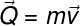Q – momentum (kg.m/s)

m – body mass (kg)

v – speed (m/s)

## Conservation of momentum

Conservation of momentum is a physical principle and says that, neglecting the effect of dissipative forces , such as friction or drag forces, the initial momentum of a body or system of bodies must equal the quantity of final move. This implies that the sum of the mass and velocity of all particles must be constant. The principle of conservation of momentum is illustrated in the idealized situation.

A in B – masses of bodies A and B

A and v B – velocities of bodies A and B before the collision

v’ A and v’ B – velocities of bodies A and B after the collision

In the figure, it is possible to observe two trucks, of masses m A in B , moving, respectively, to the left and to the right. After the collision, the trucks have the direction of their motion reversed , but continue to travel with the same speed, in module. This indicates that the total momentum was conserved , so we say that the collision between these trucks was perfectly elastic .

The same situation applies to the following example. In it we see two trains of masses equal to 30 T (30,000 kg) and 10 T (10,000 kg) that are at 10 m/s and at rest , respectively. After the collision, the 30-T train continues to move to the left , however the train, which was at rest, is moving with a speed of 15 m/s.

The verification of conservation of momentum can be done by adding the products of the mass and the speed of each of the trains. Watch:

If the condition of conservation of momentum is not respected, then part of the energy present in bodies before any phenomenon will be dissipated, that is, it will be transformed into other forms of energy, such as thermal energy , vibrations, among others. In this case, we say that an inelastic collision has occurred .

## Impulse and quantity and motion

Impulse and momentum are physical quantities that have the same unit of measurement and are related to each other. According to the impulse theorem , the change in momentum is equivalent to the impulse exerted on a body. The formula shown below relates these two quantities.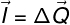I – impulse (kg.m/s)

ΔQ – variation of the momentum (kg.m/s)

## Momentum and kinetic energy

Momentum and kinetic energy (E C ) are important quantities for the study of Dynamics. The following formula shows the relationship between them: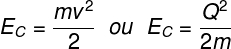## Exercises solved on momentum

Question 1 – About the physical quantity known as momentum, the following statements are made:

I – Momentum is a scalar physical quantity.

II – The unit of measure of the momentum is the kg.m/s.

III – The momentum is defined by the product of mass and velocity.

Are true :

a) I and II

b) II and III

c) I, II and III

d) Only I

e) Only III

Template: letter B.

Resolution:

Momentum can be calculated by multiplying a body’s mass by its velocity. This physical quantity is vector, and its unit of measurement, according to the SI, is kg.m/s. Therefore, statements II and III are correct .

Question 2 – A body of mass m moves with speed v . Knowing that the magnitude of the momentum of this body is equal to Q and that its kinetic energy is E , determine, in terms of Q and E , what must be the magnitudes of the momentum and kinetic energy of this same body if the velocity v was duplicated .

a) Q’ = 4Q and E C ‘ = E C /2

b) Q’ = 2Q and E C ‘ = 2E C

c) Q’ = Q/2 and E C ‘ = 4E C

d) Q’ = 2Q and E C ‘ = 4E C

e) Q’ = Q and E C ‘ = 4E C

Template : letter C.

Resolution:

To solve this question, it is necessary that we use the formulas for momentum and kinetic energy Also, we must take into account that the new speed of the body is 2v.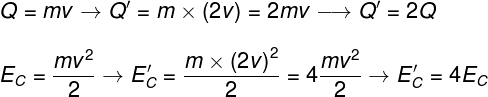Based on the calculations, we can say that the momentum is doubled, while the kinetic energy increases by four times, so the correct alternative is the letter D.

Question 3) Determine the magnitude of the momentum of a 900 kg motor vehicle traveling with a speed of 72 km/h and choose the correct alternative:

a) 1800 kg.m/s

b) 19,600 kg.m/s

c) 64,800 kg.m/s

d) 8,000 kg.m/s

e) 18,000 kg.m/s

Template: letter E.

Resolution :

In order to calculate the magnitude of the momentum, it is necessary to multiply the mass of the body by its velocity, but it is also necessary that the measurement units are defined according to the SI units. In this way, you need to divide the speed, which is in km/h, by 3.6. Check the calculation: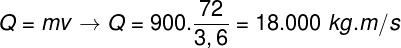Based on the calculation made, which resulted in a momentum of 18,000 kg.m/s, the correct alternative is the letter E.

Check Also
Close